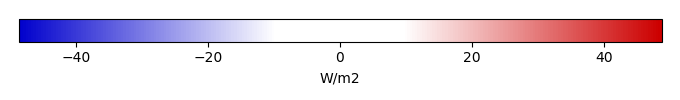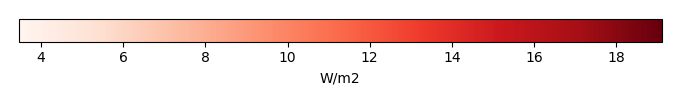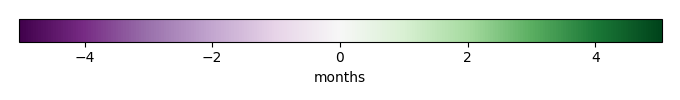# Mean State

Period Mean (original grids) [W/m2]
Model Period Mean (intersection) [W/m2]
Model Period Mean (complement) [W/m2]
Benchmark Period Mean (intersection) [W/m2]
Benchmark Period Mean (complement) [W/m2]
Bias [W/m2]
RMSE [W/m2]
Phase Shift [months]
Bias Score 
RMSE Score 
Seasonal Cycle Score 
Spatial Distribution Score 
Interannual Variability Score 
Overall Score 
Benchmark [-] 131.
CLM5PHSOFF [-] 115. 115. 0.00 130. 157. -14.2 23.1 1.40 0.50 0.42 0.82 0.99 0.71 0.64
CLM5PHSON [-] 116. 116. 0.00 130. 157. -13.7 22.6 1.40 0.51 0.42 0.81 0.99 0.69 0.64
Period Mean (original grids) [W/m2]
Model Period Mean (intersection) [W/m2]
Model Period Mean (complement) [W/m2]
Benchmark Period Mean (intersection) [W/m2]
Benchmark Period Mean (complement) [W/m2]
Bias [W/m2]
RMSE [W/m2]
Phase Shift [months]
Bias Score 
RMSE Score 
Seasonal Cycle Score 
Spatial Distribution Score 
Interannual Variability Score 
Overall Score 
Benchmark [-] 123.
CLM5PHSOFF [-] 96.1 95.6 0.00 122. 160. -26.2 31.5 1.41 0.35 0.50 0.79 0.92 0.70 0.63
CLM5PHSON [-] 97.4 96.9 0.00 122. 160. -24.8 30.3 1.43 0.37 0.50 0.79 0.94 0.65 0.63
Period Mean (original grids) [W/m2]
Model Period Mean (intersection) [W/m2]
Model Period Mean (complement) [W/m2]
Benchmark Period Mean (intersection) [W/m2]
Benchmark Period Mean (complement) [W/m2]
Bias [W/m2]
RMSE [W/m2]
Phase Shift [months]
Bias Score 
RMSE Score 
Seasonal Cycle Score 
Spatial Distribution Score 
Interannual Variability Score 
Overall Score 
Benchmark [-] 123.
CLM5PHSOFF [-] 104. 104. 0.00 121. 157. -17.7 25.7 1.10 0.57 0.60 0.85 0.94 0.73 0.71
CLM5PHSON [-] 105. 105. 0.00 121. 157. -15.0 25.1 1.49 0.62 0.57 0.77 0.96 0.68 0.69
Period Mean (original grids) [W/m2]
Model Period Mean (intersection) [W/m2]
Model Period Mean (complement) [W/m2]
Benchmark Period Mean (intersection) [W/m2]
Benchmark Period Mean (complement) [W/m2]
Bias [W/m2]
RMSE [W/m2]
Phase Shift [months]
Bias Score 
RMSE Score 
Seasonal Cycle Score 
Spatial Distribution Score 
Interannual Variability Score 
Overall Score 
Benchmark [-] 34.4
CLM5PHSOFF [-] -2.15 28.9 0.00 34.5 33.0 -5.96 21.4 0.284 0.84 0.72 0.98 0.83 0.73 0.81
CLM5PHSON [-] 32.9 33.0 0.00 34.5 33.0 -3.11 20.8 0.266 0.86 0.72 0.98 0.82 0.74 0.81
Period Mean (original grids) [W/m2]
Model Period Mean (intersection) [W/m2]
Model Period Mean (complement) [W/m2]
Benchmark Period Mean (intersection) [W/m2]
Benchmark Period Mean (complement) [W/m2]
Bias [W/m2]
RMSE [W/m2]
Phase Shift [months]
Bias Score 
RMSE Score 
Seasonal Cycle Score 
Spatial Distribution Score 
Interannual Variability Score 
Overall Score 
Benchmark [-] 147.
CLM5PHSOFF [-] 133. 132. 0.00 145. 156. -12.6 21.7 1.65 0.48 0.43 0.74 0.99 0.69 0.63
CLM5PHSON [-] 128. 127. 0.00 145. 156. -13.0 21.7 1.63 0.48 0.43 0.74 0.99 0.69 0.63
Period Mean (original grids) [W/m2]
Model Period Mean (intersection) [W/m2]
Model Period Mean (complement) [W/m2]
Benchmark Period Mean (intersection) [W/m2]
Benchmark Period Mean (complement) [W/m2]
Bias [W/m2]
RMSE [W/m2]
Phase Shift [months]
Bias Score 
RMSE Score 
Seasonal Cycle Score 
Spatial Distribution Score 
Interannual Variability Score 
Overall Score 
Benchmark [-] 53.7
CLM5PHSOFF [-] 42.8 42.7 0.00 53.5 64.9 -10.8 19.7 0.208 0.82 0.76 0.99 0.94 0.72 0.83
CLM5PHSON [-] 48.1 48.1 0.00 53.5 64.9 -5.14 17.0 0.214 0.87 0.77 0.99 0.90 0.72 0.84
Period Mean (original grids) [W/m2]
Model Period Mean (intersection) [W/m2]
Model Period Mean (complement) [W/m2]
Benchmark Period Mean (intersection) [W/m2]
Benchmark Period Mean (complement) [W/m2]
Bias [W/m2]
RMSE [W/m2]
Phase Shift [months]
Bias Score 
RMSE Score 
Seasonal Cycle Score 
Spatial Distribution Score 
Interannual Variability Score 
Overall Score 
Benchmark [-] 71.7
CLM5PHSOFF [-] 62.1 62.0 0.00 71.1 90.6 -9.09 19.7 0.442 0.79 0.72 0.96 0.99 0.70 0.81
CLM5PHSON [-] 62.6 62.6 0.00 71.1 90.6 -7.26 19.0 0.429 0.81 0.72 0.96 0.99 0.71 0.82
Period Mean (original grids) [W/m2]
Model Period Mean (intersection) [W/m2]
Model Period Mean (complement) [W/m2]
Benchmark Period Mean (intersection) [W/m2]
Benchmark Period Mean (complement) [W/m2]
Bias [W/m2]
RMSE [W/m2]
Phase Shift [months]
Bias Score 
RMSE Score 
Seasonal Cycle Score 
Spatial Distribution Score 
Interannual Variability Score 
Overall Score 
Benchmark [-] 120.
CLM5PHSOFF [-] 97.2 96.5 0.00 118. 153. -21.4 28.8 0.658 0.51 0.56 0.92 0.99 0.66 0.70
CLM5PHSON [-] 95.0 94.6 0.00 118. 153. -18.8 27.5 0.690 0.55 0.54 0.92 0.99 0.65 0.70
Period Mean (original grids) [W/m2]
Model Period Mean (intersection) [W/m2]
Model Period Mean (complement) [W/m2]
Benchmark Period Mean (intersection) [W/m2]
Benchmark Period Mean (complement) [W/m2]
Bias [W/m2]
RMSE [W/m2]
Phase Shift [months]
Bias Score 
RMSE Score 
Seasonal Cycle Score 
Spatial Distribution Score 
Interannual Variability Score 
Overall Score 
Benchmark [-] 30.5
CLM5PHSOFF [-] 21.8 22.2 0.00 31.0 19.5 -8.80 19.8 0.202 0.84 0.74 0.99 0.98 0.74 0.84
CLM5PHSON [-] 24.2 24.5 0.00 31.0 19.5 -7.63 19.3 0.205 0.86 0.74 0.99 0.98 0.75 0.84
Period Mean (original grids) [W/m2]
Model Period Mean (intersection) [W/m2]
Model Period Mean (complement) [W/m2]
Benchmark Period Mean (intersection) [W/m2]
Benchmark Period Mean (complement) [W/m2]
Bias [W/m2]
RMSE [W/m2]
Phase Shift [months]
Bias Score 
RMSE Score 
Seasonal Cycle Score 
Spatial Distribution Score 
Interannual Variability Score 
Overall Score 
Benchmark [-] 109.
CLM5PHSOFF [-] 94.0 93.9 0.00 108. 131. -13.8 20.9 0.505 0.73 0.73 0.96 0.95 0.80 0.81
CLM5PHSON [-] 93.6 93.6 0.00 108. 131. -12.2 20.1 0.514 0.75 0.73 0.96 0.96 0.77 0.82
Period Mean (original grids) [W/m2]
Model Period Mean (intersection) [W/m2]
Model Period Mean (complement) [W/m2]
Benchmark Period Mean (intersection) [W/m2]
Benchmark Period Mean (complement) [W/m2]
Bias [W/m2]
RMSE [W/m2]
Phase Shift [months]
Bias Score 
RMSE Score 
Seasonal Cycle Score 
Spatial Distribution Score 
Interannual Variability Score 
Overall Score 
Benchmark [-] 65.7
CLM5PHSOFF [-] 54.8 54.7 0.00 63.8 85.4 -9.07 18.1 0.215 0.85 0.78 0.99 0.99 0.67 0.84
CLM5PHSON [-] 54.7 54.7 0.00 63.8 85.4 -6.96 17.5 0.197 0.87 0.78 0.99 0.99 0.68 0.85
Period Mean (original grids) [W/m2]
Model Period Mean (intersection) [W/m2]
Model Period Mean (complement) [W/m2]
Benchmark Period Mean (intersection) [W/m2]
Benchmark Period Mean (complement) [W/m2]
Bias [W/m2]
RMSE [W/m2]
Phase Shift [months]
Bias Score 
RMSE Score 
Seasonal Cycle Score 
Spatial Distribution Score 
Interannual Variability Score 
Overall Score 
Benchmark [-] 109.
CLM5PHSOFF [-] 72.3 65.1 0.00 80.5 123. -15.5 23.9 0.654 0.62 0.62 0.92 0.98 0.69 0.74
CLM5PHSON [-] 65.1 65.1 0.00 80.5 123. -13.7 23.0 0.692 0.64 0.61 0.92 0.98 0.68 0.74
Period Mean (original grids) [W/m2]
Model Period Mean (intersection) [W/m2]
Model Period Mean (complement) [W/m2]
Benchmark Period Mean (intersection) [W/m2]
Benchmark Period Mean (complement) [W/m2]
Bias [W/m2]
RMSE [W/m2]
Phase Shift [months]
Bias Score 
RMSE Score 
Seasonal Cycle Score 
Spatial Distribution Score 
Interannual Variability Score 
Overall Score 
Benchmark [-] 76.6
CLM5PHSOFF [-] 55.2 55.1 0.00 73.9 144. -18.8 25.0 0.885 0.55 0.62 0.90 0.93 0.70 0.72
CLM5PHSON [-] 53.6 53.6 0.00 73.9 144. -18.0 24.8 0.888 0.56 0.62 0.90 0.94 0.71 0.72
Period Mean (original grids) [W/m2]
Model Period Mean (intersection) [W/m2]
Model Period Mean (complement) [W/m2]
Benchmark Period Mean (intersection) [W/m2]
Benchmark Period Mean (complement) [W/m2]
Bias [W/m2]
RMSE [W/m2]
Phase Shift [months]
Bias Score 
RMSE Score 
Seasonal Cycle Score 
Spatial Distribution Score 
Interannual Variability Score 
Overall Score 
Benchmark [-] 36.2
CLM5PHSOFF [-] 24.1 23.9 0.00 36.2 36.3 -12.3 22.1 0.0876 0.80 0.73 0.99 0.95 0.77 0.83
CLM5PHSON [-] 27.4 27.3 0.00 36.2 36.3 -10.9 21.5 0.0841 0.82 0.73 0.99 0.93 0.77 0.83
Period Mean (original grids) [W/m2]
Model Period Mean (intersection) [W/m2]
Model Period Mean (complement) [W/m2]
Benchmark Period Mean (intersection) [W/m2]
Benchmark Period Mean (complement) [W/m2]
Bias [W/m2]
RMSE [W/m2]
Phase Shift [months]
Bias Score 
RMSE Score 
Seasonal Cycle Score 
Spatial Distribution Score 
Interannual Variability Score 
Overall Score 
Benchmark [-] 138.
CLM5PHSOFF [-] 110. 110. 0.00 133. 161. -22.3 29.0 1.06 0.53 0.62 0.87 0.99 0.72 0.72
CLM5PHSON [-] 107. 107. 0.00 133. 161. -20.2 27.5 1.29 0.56 0.62 0.83 0.99 0.70 0.72
Period Mean (original grids) [W/m2]
Model Period Mean (intersection) [W/m2]
Model Period Mean (complement) [W/m2]
Benchmark Period Mean (intersection) [W/m2]
Benchmark Period Mean (complement) [W/m2]
Bias [W/m2]
RMSE [W/m2]
Phase Shift [months]
Bias Score 
RMSE Score 
Seasonal Cycle Score 
Spatial Distribution Score 
Interannual Variability Score 
Overall Score 
Benchmark [-] 98.6
CLM5PHSOFF [-] 80.1 79.9 0.00 96.9 135. -16.9 22.4 0.478 0.73 0.76 0.96 0.95 0.73 0.82
CLM5PHSON [-] 81.8 81.9 0.00 96.9 135. -13.3 19.7 0.468 0.78 0.76 0.96 0.93 0.73 0.82
Period Mean (original grids) [W/m2]
Model Period Mean (intersection) [W/m2]
Model Period Mean (complement) [W/m2]
Benchmark Period Mean (intersection) [W/m2]
Benchmark Period Mean (complement) [W/m2]
Bias [W/m2]
RMSE [W/m2]
Phase Shift [months]
Bias Score 
RMSE Score 
Seasonal Cycle Score 
Spatial Distribution Score 
Interannual Variability Score 
Overall Score 
Benchmark [-] 63.9
CLM5PHSOFF [-] 53.2 53.4 0.00 63.6 72.9 -10.3 18.5 0.149 0.83 0.78 0.99 0.99 0.72 0.85
CLM5PHSON [-] 58.2 58.3 0.00 63.6 72.9 -5.21 15.3 0.153 0.90 0.79 0.99 0.99 0.71 0.86
Period Mean (original grids) [W/m2]
Model Period Mean (intersection) [W/m2]
Model Period Mean (complement) [W/m2]
Benchmark Period Mean (intersection) [W/m2]
Benchmark Period Mean (complement) [W/m2]
Bias [W/m2]
RMSE [W/m2]
Phase Shift [months]
Bias Score 
RMSE Score 
Seasonal Cycle Score 
Spatial Distribution Score 
Interannual Variability Score 
Overall Score 
Benchmark [-] 82.3
CLM5PHSOFF [-] 68.6 68.8 0.00 81.8 110. -13.1 20.1 0.526 0.78 0.76 0.95 0.94 0.74 0.82
CLM5PHSON [-] 71.4 71.6 0.00 81.8 110. -9.78 18.5 0.542 0.81 0.77 0.95 0.95 0.74 0.83
Period Mean (original grids) [W/m2]
Model Period Mean (intersection) [W/m2]
Model Period Mean (complement) [W/m2]
Benchmark Period Mean (intersection) [W/m2]
Benchmark Period Mean (complement) [W/m2]
Bias [W/m2]
RMSE [W/m2]
Phase Shift [months]
Bias Score 
RMSE Score 
Seasonal Cycle Score 
Spatial Distribution Score 
Interannual Variability Score 
Overall Score 
Benchmark [-] 139.
CLM5PHSOFF [-] 121. 121. 0.00 138. 170. -16.7 24.5 1.09 0.35 0.40 0.85 0.98 0.74 0.62
CLM5PHSON [-] 121. 122. 0.00 138. 170. -15.8 24.0 1.23 0.36 0.40 0.82 0.97 0.72 0.61
Period Mean (original grids) [W/m2]
Model Period Mean (intersection) [W/m2]
Model Period Mean (complement) [W/m2]
Benchmark Period Mean (intersection) [W/m2]
Benchmark Period Mean (complement) [W/m2]
Bias [W/m2]
RMSE [W/m2]
Phase Shift [months]
Bias Score 
RMSE Score 
Seasonal Cycle Score 
Spatial Distribution Score 
Interannual Variability Score 
Overall Score 
Benchmark [-] 121.
CLM5PHSOFF [-] 105. 105. 0.00 120. 143. -15.5 24.0 0.868 0.63 0.61 0.91 0.84 0.74 0.72
CLM5PHSON [-] 107. 107. 0.00 120. 143. -12.8 22.6 0.892 0.66 0.61 0.90 0.82 0.70 0.72
Period Mean (original grids) [W/m2]
Model Period Mean (intersection) [W/m2]
Model Period Mean (complement) [W/m2]
Benchmark Period Mean (intersection) [W/m2]
Benchmark Period Mean (complement) [W/m2]
Bias [W/m2]
RMSE [W/m2]
Phase Shift [months]
Bias Score 
RMSE Score 
Seasonal Cycle Score 
Spatial Distribution Score 
Interannual Variability Score 
Overall Score 
Benchmark [-] 98.1
CLM5PHSOFF [-] 88.4 87.6 0.00 95.8 118. -8.41 24.3 0.514 0.75 0.70 0.95 0.99 0.64 0.79
CLM5PHSON [-] 90.4 89.8 0.00 95.8 118. -7.08 23.4 0.560 0.76 0.71 0.95 0.99 0.64 0.79

# Temporally integrated period mean

BENCHMARK MEANMODEL MEANBIASBIAS SCORERMSERMSE SCOREBENCHMARK INTERANNUAL VARIABILITYMODEL INTERANNUAL VARIABILITYINTERANNUAL VARIABILITY SCOREBENCHMARK MAX MONTHMODEL MAX MONTHDIFFERENCE IN MAX MONTHSEASONAL CYCLE SCORESPATIAL TAYLOR DIAGRAMMODEL COLORS# Spatially integrated regional mean

MODEL COLORSREGIONAL MEANANNUAL CYCLEMONTHLY ANOMALYANNUAL CYCLE# All Models

BenchmarkCLM5PHSOFFCLM5PHSON# Data Information

creation_date: Thu May 8 22:58:12 PDT 2014

source_file: This product is generated from monthly 1 degree CERES EBAF Radiation observations